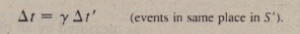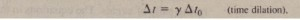# Time Dilation

Time Dilation

Suppose now that two events occur at the same place in S’ (so .1x’ = 0) but at different times (so .1(‘ “* 0). Equation 38-22 then reduces toThis confirms time dilation. Because the two events occur at the same place in S’, the time interval t!.(‘ between them can be measured with a single clock, located at that place. Under these conditions, the measured interval is a proper time interval, and we can label it .1(0′ Thus, Eq. 38-23 becomeswhich is exactly Eq. 38-9. the time dilation equation.